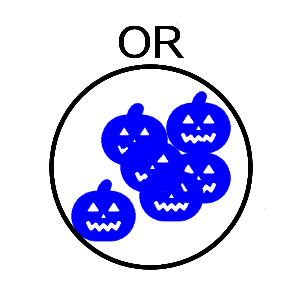## Fun With SAS ODS Graphics - Halloween 2020 Electoral MapHalloween 2020 Electoral Map

To mark Halloween 2020, here are the 10-31 predicted electoral votes from CNN presented in a cartogram scatter plot of jittered pumpkin markers from the Noun Project together with a bar chart summary. Happy Halloween!

SAS CODE

``````* Fun With SAS ODS Graphics - Halloween 2020 Electoral Map
Data courtesy of CNN - cnn.com/election/2020/electoral-college-interactive-maps
Pumpkin icon courtesy of Saepul Nahwan - thenounproject.com/term/halloween-pumpkin/3435605/;

*==> Get CNN.com state-level election prediction data (JSON format);

filename cnn '/folders/myfolders/CNN2020JSON20201031.txt';
libname j json fileref=cnn;
proc sql;
create table CNNpredictions as
case when v.party='Dem' & v.type='win' then 'WIN (D)' when v.party='Dem' & v.type='lean' then 'LEAN (D)'
when v.party='Rep' & v.type='win' then 'WIN (R)' when v.party='Rep' & v.type='lean' then 'LEAN (R)'
when v.party='Tossup' then 'TOSSUP' else trim(v.party)||' '||v.type
end as Prediction
from j.root j join j.electoralvotes v on j.ordinal_root=v.ordinal_root
order by statecode, prediction;

*==> Generate USA cartogram map x/y coordinates from inline layout of state codes;

data states(keep=statecode x y y2);
input states \$char80.;
y+1;
y2=y-.47;                            * y-axis location of state name;
x=mod(y-1,2)*.5;                     * Offset alternate rows of states by .5;
do c=1+mod(y-1,2)*2 to 80 by 4;
statecode=substr(states,c,2);
x+1;
if statecode^='' then output;
end;
datalines;
AK                                          ME
VT, NH
WA, MT, ND, MN, WI,     MI,     NY, MA, RI
ID, WY, SD, IA, IL, IN, OH, PA, NJ, CT
OR, NV, CO, NE, MO, KY, WV, MD, DE
CA, AZ, UT, KS, AR, TN, VA, NC,         DC
NM, OK, LA, MS, AL, SC
TX              GA
HI                              FL
;
proc sort data=states; by statecode;

*==> Create one observation per electoral vote, add sort seq;

merge states cnnpredictions;
by statecode;                       * Assign sort sequence for bar chart;
sortseq=index('WIN (D), LEAN (D), TOSSUP, LEAN (R), WIN (R)', trim(prediction));
dummyvar='D';
output;
end;

proc sort data=statesvotes; by sortseq; * Order for bar chart;

*==> Map and bar charts of predicted voting results (Scatter+Ellipse+Text+Bar plots);

ods listing image_dpi=300 gpath='/folders/myfolders';
ods graphics on / reset antialias width=11in height=8.5in imagename="PresidentialElection";

proc template;
define statgraph ustemplate;
begingraph / border=true;         * Define Dem/Rep color and image attributes;
symbolimage name=symPLD image="/folders/myfolders/PumpkinPaleBlue.png";
symbolimage name=symPWD image="/folders/myfolders/PumpkinBlue.png";
symbolimage name=symPLR image="/folders/myfolders/PumpkinLightRed.png";
symbolimage name=symPWR image="/folders/myfolders/PumpkinRed.png";
symbolimage name=symPTU image="/folders/myfolders/PumpkinOrange.png";
value "LEAN (D)" / markerattrs=(symbol=symPLD size=16pt) fillattrs=(color=cxA3A3FF);
value "WIN (D)" / markerattrs=(symbol=symPWD size=16pt) fillattrs=(color=cx0000ff);
value "LEAN (R)" / markerattrs=(symbol=symPLR size=16pt) fillattrs=(color=cxf1959b);
value "WIN (R)" / markerattrs=(symbol=symPWR size=16pt) fillattrs=(color=cxff0000);
value "TOSSUP" / markerattrs=(symbol=symPTU size=16pt) fillattrs=(color=cxFF9900);
enddiscreteattrmap;              * Define custom legend for markers;
discreteattrvar attrvar=groupmarkers var=prediction attrmap="symbols";
legendItem type=marker name="pwd" / markerattrs=(symbol=symPWD size=28pt) label="WIN (D)" labelattrs=(size=9pt weight=bold);
legendItem type=marker name="pld" / markerattrs=(symbol=symPLD size=28pt) label="LEAN (D)" labelattrs=(size=9pt weight=bold);
legendItem type=marker name="ptu" / markerattrs=(symbol=symPTU size=28pt) label="TOSSUP" labelattrs=(size=9pt weight=bold);
legendItem type=marker name="plr" / markerattrs=(symbol=symPLR size=28pt) label="LEAN (R)" labelattrs=(size=9pt weight=bold);
legendItem type=marker name="pwr" / markerattrs=(symbol=symPWR size=28pt) label="WIN (R)" labelattrs=(size=9pt weight=bold);

layout lattice / border=false rows=2 rowweights=(0.95 0.05);   * Cartogram map + scatterplot of all electoral votes;

layout overlayequated / border=false xaxisopts=(display=none) yaxisopts=(display=none reverse=true) walldisplay=none;
entry "Halloween 2020 Electoral Map (CNN 10/31 Data)" / valign=top textattrs=(size=16pt weight=bold);
scatterplot x=x y=y /  jitter=auto jitteropts=(width=1) group=groupmarkers;
ellipseparm semiminor=.48 semimajor=.48 xorigin=x yorigin=y slope=0 / outlineattrs=(color=black);
textplot x=x y=y2 text=statecode /  position=top textattrs=(color=black size=9pt);
discretelegend "pwd" "pld" "ptu" "plr" "pwr" / location=inside border=false valign=bottom;
endlayout;
* Bar chart summary of electoral votes;
layout overlay / border=false xaxisopts=(display=none) yaxisopts=(display=none reverse=true) walldisplay=none;
barchart category=dummyvar / stat=freq display=(fill) displaybaseline=off group=groupmarkers groupdisplay=stack orient=horizontal segmentlabel=true segmentlabelattrs=(size=10pt color=white weight=bold);
referenceline x=270 / lineattrs=(color=white);               * Takes 270 votes to win!;
endlayout;

endlayout;
endgraph;
end;
run;

proc sgrender data=statesvotes template=ustemplate;              * Generate the charts!;``````

STATE DETAILOregon Detail

CNN JSON Snippet

`[{"stateCode":"AL","type":"win","party":"Rep","electoralVotes":[{"type":"win","party":"Rep","electoralVotes":9}]},{"stateCode":"AK","type":"win","party":"Rep","electoralVotes":[{"type":"win","party":"Rep","electoralVotes":3}]},{"stateCode":"AZ","type":"lean","party":"Dem","battleground":true,"electoralVotes":[{"type":"lean","party":"Dem","electoralVotes":11}]},...`
2 REPLIES 2

## Re: Fun With SAS ODS Graphics - Halloween 2020 Electoral Map

Thanks a bunch for sharing the ebullient graph!

I tried to play your SAS code but have a difficulty to get input file named "CNN2020JSON20201031.txt". Could you shed some lights on it?

Thx.

Ethan

## Re: Fun With SAS ODS Graphics - Halloween 2020 Electoral Map

Sure, it was just the 2020 state prediction data from CNN. To obtain the data, I did a "View Source" on the web page at https://www.cnn.com/election/2020/electoral-college-interactive-maps on 10/31, located the JSON data for the map, and simply copied-and-pasted the state entries into a text file, which I've uploaded (see attached file). Good luck!

Discussion stats
• 2 replies
• 733 views
• 2 likes
• 2 in conversation